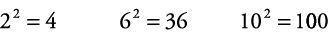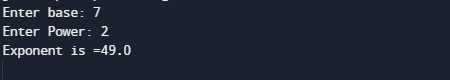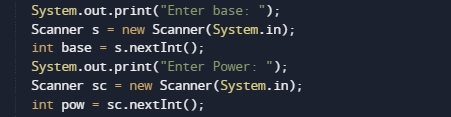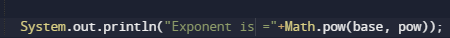# Java Program to Find exponents

In this tutorial you will learn about the Java Program to Find exponents and its application with practical example.

In this tutorial, we will learn to create a Java Program to find Exponent using Java programming.

## Prerequisites

Before starting with this tutorial we assume that you are best aware of the following Java programming topics:

• Java Operators.
• Basic Input and Output function in Java.
• Class and Object in Java.
• Basic Java programming.
• If-else statements in Java.
• For loop in Java.
• In-built functions.

## Exponent of a Number

Let’s take a value at  base : 2
Now take a value at exponent : 3
So power(Exponent) of any number is basen given as base*base*base (3-times). Here base is called base and 3 is called the exponent.are some example of exponents.

## Java Program to Find exponents

In this program we will find exponent of any given number by user using  loop. We would first declared and initialized the required variables. Next, we would prompt user to input the number and power of number .Then we will find the power of given number.

## OutputNote : The above program works only if the Power is a positive integer (number).

In the above program, we have first declared and initialized a set variables required in the program.

• base = it hold the value of base
• pow = it will hold the power.

Let see the logic behind the the program

• Take value of  “base and exponents(Power)” from user, and put  it in two variables  base and pow respectively.Here we will to use the “Math.pow(base, pow))” function which is define  in Java standard library.it will take two parameter as a arguments base and power and returns the exponent of value supply to it.At last print the resultant output as shown in image above.

In this tutorial we have learn about the Java Program to Find exponents and its application with practical example. I hope you will like this tutorial.